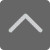## 爱智康资讯站

### 课程咨询: 4000-121-121

• 全国课程在线咨询
• 咨询热线：4000-121-121
• 登录手机官网

• 关注微信公众号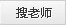# 加减乘除速算法，为孩子打开一个神奇有趣的数学世界！

2020-05-12 16:59:46 　来源：西安爱智康 文章作者：西安爱智康

## 学而思・爱智康1对1/8人班 帮助孩子解决知识难点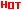*2470人已预约，首课体验不满意，可全额退费。

― ― 报名课程可获得 ― ―

1、口诀

2、例题

1376+98=1474 计算方法：1376+100-2

3586+898=4484 计算方法：3586+1000-102

5768+9897=15665 计算方法：5768+10000-103

1、口诀

2、例题

47+74=121 计算方法：（4+7）x 11=121

68+86=154 计算方法：（6+8）x 11=154

58+85=143 计算方法：（5+8）x 11=143

1、例题

321-98=223

8135-878=7257

91321-8987= 82334

2、总结

1、例题

74-47=27

83-38=45

92-29=63

2、总结

1、例题

936-639=297

723-327=396

873-378=495

2、总结

1、例题

73-27=46

613-387=226

8112-1888=6224

2、总结

1、口诀

2、例题

67x 63= 4221

7x3=21写在42的后面，即为乘积4221

38x32=1216

8x2=16写在12的后面，即为乘积1216

76x74=5624

6x4=24写在56的后面，即为乘积5624

81 x89=7209

1x9=09写在72的后面，（未满10补零）即为乘积7209

1.口诀

2.例题

76x 36＝2736

6x6= 36写在27的后面，即乘积2736

68x 48＝3264

8x8=64写在32的后面，即为乘积3264

57的平方是5x5+7+7x7=3249

........

1、例题

37x66=2442

7x6=42写在24的后面，即乘积2442

44x28=1232

4x8=32写在12的后面，即乘积1232

2、总结

1、例题

13x12=156

3x2=6 150+6=156

15x17=255

5x7=35 220+35=255

2、口诀

1、例题

31x21=651

51 x71=3621

5+7=12（写2进1） 1x1=1

61 x81=4941

6+8=14（写4进1） 1x1=1

2、口诀

1、例题

101Ｘ102=10302

1Ｘ2=02 两数相接即为乘积10302

103 Ｘ104=10712

3Ｘ4=12

2、口诀

1、例题

7995÷65=123，(65的补数是35)

2、算序

①被除数前两位79中含除数65一倍，加补数一次(35)，得1-1495(破折号前为商，破折号后为被除数，下同);

②被乘数149中含除数二倍，加补数二次(35×2=70)得12-195;

③被除数195含除数三倍，加补数三次(35×3=105)得123(商)。

1、例题

35568÷78=456(78的补数是22)

2、算序

355中含有除数4倍，所以前位加11，本位减22，得4-4368;

436中含除数5倍，前位加11，本位不动，得45-468;

468中含除数6倍，前位加11，本位加22，得456(商)。

1、例题

884352÷896=987(896的补数是104)

2、算序

①8843中含除数9倍，前位加104，本位减104，得9-77952;

②7795中含除数8倍前位加104，本位减208，得98-6272;

③6272含除数7倍，前位加补数一次104，本位减补数三次(104×3=312(得986(商))。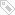标签：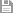保存　｜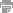打印　｜关闭